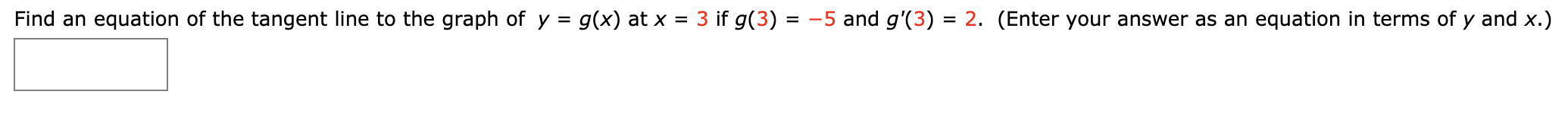# Find an equation of the tangent line to the graph of y = g(x) at x = 3 if g(3) = -5 and g'(3) = 2. (Enter your answer as an equation in terms of y and x.)

Question
1 viewshelp_outlineImage TranscriptioncloseFind an equation of the tangent line to the graph of y = g(x) at x = 3 if g(3) = -5 and g'(3) = 2. (Enter your answer as an equation in terms of y and x.) fullscreen
check_circle

Step 1

Here, y = g(x)  and g(3) = -5

i.e. when x1 = 3, then  y1 = -5

and g(3)=2

i.e. At x =3, the slope, m = 2

Thus, (y -y1) = m(x - x1)

Putting the ...

### Want to see the full answer?

See Solution

#### Want to see this answer and more?

Solutions are written by subject experts who are available 24/7. Questions are typically answered within 1 hour.*

See Solution
*Response times may vary by subject and question.
Tagged in

### Calculus